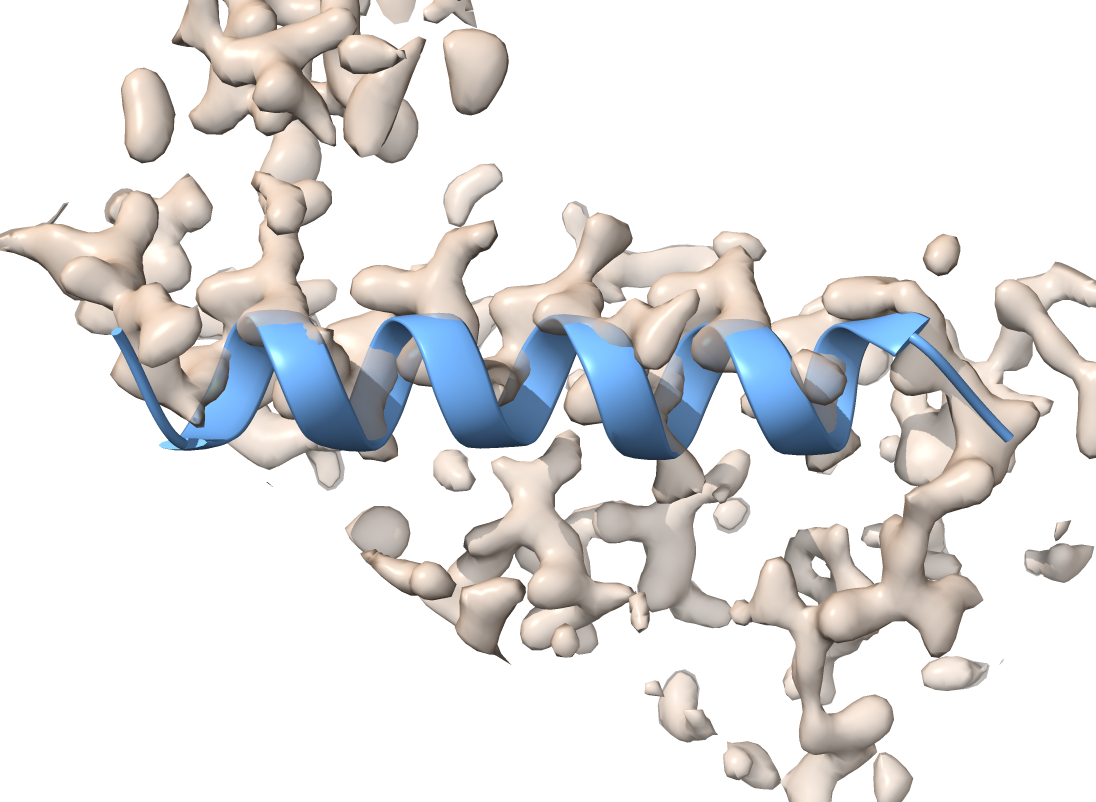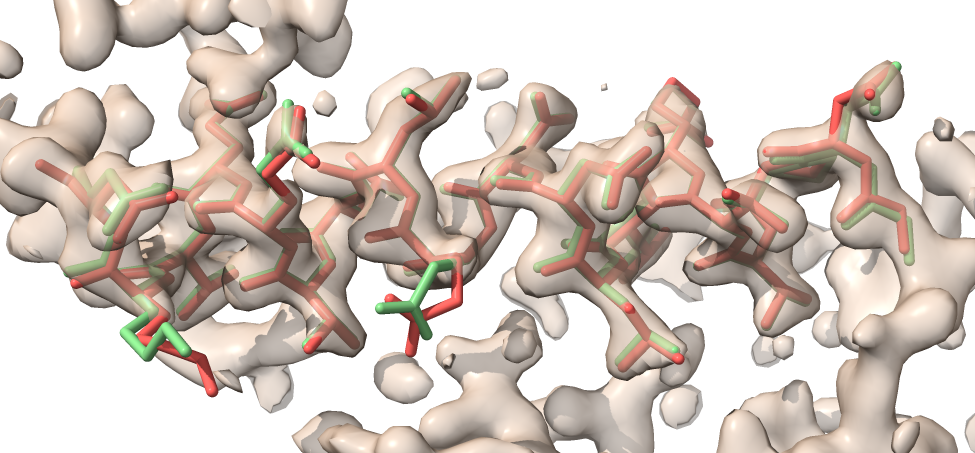## Morphing a model

Learn how to adjust a model by morphing it to match a map. Refinement can further improve the model-to-map fit.

### Setting up example data

First, let's set up the example data (described in more detail here):

Get the files from the Phenix regression directory and change into the new folder:

``````phenix.setup_tutorial tutorial_name=model-building-scripting
cd model-building-scripting``````

Type `phenix.python` to start up Python with the Phenix environment all set up:

``phenix.python``

Set up the high level objects, so we can start our task:

``````from iotbx.data_manager import DataManager    # Load in the DataManager
dm = DataManager()             # Initialize the DataManager and call it dm
dm.set_overwrite(True)         # Overwrite files with the same name
mmm = dm.get_map_model_manager(        # getting a map_model_manager
model_file="short_model_box_for_morph.pdb",   # model file
map_files="short_model_box.ccp4")   # map file
``````

### Morphing a model

Let’s adjust a model by morphing it to match the density in a map. Morphing basically distorts a model in a smooth way, so that locally the model does not change much, but parts of the model that are far apart along the chain can move relative to each other.

Our starting model has two chains (A and B). The B chain is offset from its correct position by about 1.5 Å.Let’s select just chain B to work with (in this example, chains A and B overlap and so it is best to work with just one).

``````chainB = mmm.model().apply_selection_string(    # apply a selection
'chain B'  )  # chain B
``````

And now we can replace the model in mmm with chainB (adding a model with model_id of 'model' replaces the existing model):

``````mmm.add_model_by_id(chainB, model_id = 'model')  # replace model in mmm
``````

Let’s fill in the resolution and experiment type and get a model_building object:

``````mmm.set_resolution(3)                 # resolution is 3 A
mmm.set_experiment_type('cryo_em')    # it is a cryo-EM map
build = mmm.model_building()          # get a model-building object
``````

We can write out the model and look at it with Coot, comparing it with the map:

``````dm.write_model_file(build.model(), "short_model_box_for_morph.pdb") # model
dm.write_real_map_file(build.map_manager(), "short_model_box.ccp4") # map
``````

Let’s save the coordinates of the atoms in our model so we can compare them to their positions after morphing:

``````chainB = build.model().deep_copy()   # save chain B
starting_coords_chain_B = chainB.get_sites_cart()    # get coordinates chain B
``````

Now let’s morph the model. We’ll use the selection method 'by_segment' to choose how to split up the model when morphing. This choice means split up by chains, and also split any chains that are broken:

``````build.set_defaults(debug=True)      # debugging run
build.morph(default_selection_method='by_segment')   # morph model
``````

We can see how much each chain has moved. The model in build has been updated so we get the coordinates of the atoms in this working model:

``````chainB_morphed = build.model()  # chain B
final_coords_chain_B = chainB_morphed.get_sites_cart()    # get coordinates chain B
``````

The rmsd between starting and final chains A and B are then:

``````rms_B = final_coords_chain_B.rms_difference(starting_coords_chain_B)  # rms B
``````

You can print this out:

``````rms_B   # print out rms value
``````

Which yields something like:

``1.5801081738194553``

We can compare the map-model correlations of the original and morphed models:

``````cc_before = mmm.map_model_cc(model = chainB)  # map-model cc for chain B
cc_after = mmm.map_model_cc(model = chainB_morphed)  # map-model cc after
cc_before, cc_after    # cc before and after morphing
``````

Which yields something like:

``(0.25513080677867195, 0.818070481365688)``

Indicating that the map-model correlation is much higher after morphing.

Let’s write out the morphed model to 'morphed_model.pdb' and compare it in Coot with the original in "short_model_box_for_morph.pdb":

``````dm.write_model_file(build.model(), 'morphed_model.pdb')  # write out morphed
``````

The morphed model (red) is moved compared to the initial model (transparent blue), and it now fits to the density.### Refining a model

Let’s run a simple version of real-space refinement to improve the morphed model (see section above). This option is just a simplified version of phenix.real_space_refine that is suitable for quick improvement of a model while you are in the middle of model-building. For serious refinement you will want to use the standalone phenix.real_space_refine tool.

Let’s refine our morphed model from the previous section. This is pretty easy. You type:

``````build.refine()     # refine working model against working map
``````

and wait a minute or two and now you can write out the refined model:

``````dm.write_model_file(build.model(), 'refined_model.pdb')  # refined
``````

We can get the map-model correlation now:

``````cc_refined = mmm.map_model_cc(model = build.model())  # refined cc
``````

And print it out:

``````cc_refined  # print out refined cc
``````

Which gives something like:

``0.8237540703832011``

Which is just a tiny bit better than the morphed model.

Have a look at morphed_model.pdb and refined_model.pdb along with the map in short_model_box.ccp4 and you will see that the refined model (green) matches the map better than the morphed model without refinement (red).